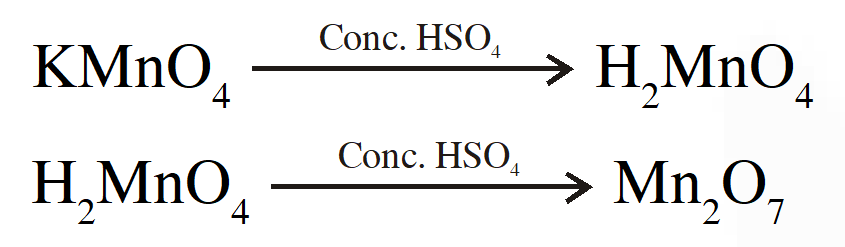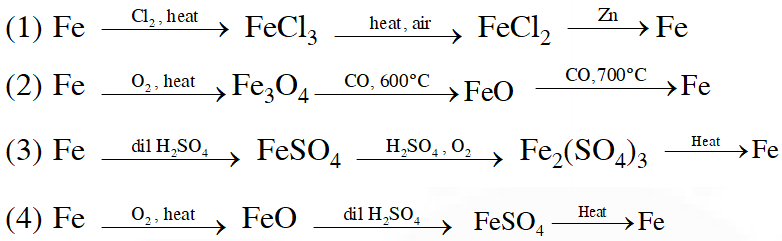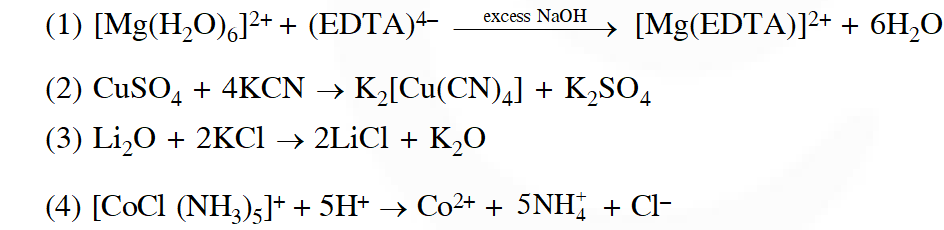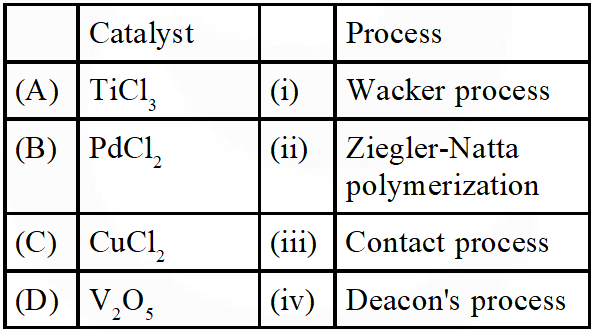Most Affordable JEE | NEET | 8,9,10 Preparation by Kota's Top IITian Doctor Faculties

# d & f - block - JEE Main Previous Year Questions with SolutionsJEE Main Previous Year Papers Questions of Chemistry with Solutions are available at eSaral. Practicing JEE Main chapter wise questions of Chemistry will help the JEE aspirants in realizing the question pattern as well as help in analyzing weak & strong areas. Simulator Previous Years AIEEE/JEE Main Question
Q. In context with the transition elements, which of the following statements is incorrect? (1) In the highest oxidation states of the first five transition elements (Sc to Mn), all the 4s and 3d electrons are used for bonding. (2) Once the d5 configuration is exceeded, the tendency to involve all the 3d electrons in bonding decreases. (3) In addition to the normal oxidation states, the zero oxidation state is also shown by these elements in complexes. (4) In the highest oxidation states, the transition metal show basic character and form cationic complexes. [AIEEE-2009]
Ans. (4)
Q. Iron exhibits +2 and +3 oxidation states. Which of the following statements about iron is incorrect ? (1) Ferrous compounds are more easily hydrolysed than the corresponding ferric compounds. (2) Ferrous oxide is more basic in nature than the ferric oxide. (3) Ferrous compounds are relatively more ionic than the corresponding ferric compounds. (4) Ferrous compounds are less volatile than the corresponding ferric compounds. [AIEEE-2012]
Ans. (1)
Q. Consider the following reaction : $\mathrm{xMnO}_{4}^{-}+\mathrm{yC}_{2} \mathrm{O}_{4}^{2-}+\mathrm{zH}^{+} \rightarrow \mathrm{xMn}^{2+}+2 \mathrm{yCO}_{2}+\frac{\mathrm{Z}}{2} \mathrm{H}_{2} \mathrm{O}$ The values of x, y and z in the reaction are respectively :- (1) 5,2 and 16 (2) 2,5 and 8 (3) 2, 5 and 16 (4) 5,2 and 8 [JEE MAIN-2013]
Ans. (3)
Q. Which of the following arrangements does not represent the correct order of the property stated against it ? (1) $\mathrm{V}^{2+}<\mathrm{Cr}^{2+}<\mathrm{Mn}^{2}<\mathrm{Fe}^{2+}$: paramagnetic behaviour (2) $\mathrm{Ni}^{2+}<\mathrm{Co}^{2+}<\mathrm{Fe}^{2+}<\mathrm{Mn}^{2+}$: ionic size (3)$\mathrm{Co}^{3+}<\mathrm{Fe}^{3+}<\mathrm{Cr}^{3+}<\mathrm{Sc}^{3+}$: stability in aqueous solution (4) $\mathrm{Sc}<\mathrm{Ti}<\mathrm{Cr}<$Mn : number of oxidation states [JEE MAIN-2013]
Ans. (1)
Q. Potassium dichromate when heated with concentrated sulphuric acid and a soluble chloride, gives brown - red vapours of: (1) $\mathrm{CrO}_{3}$ (2) $\mathrm{Cr}_{2} \mathrm{O}_{3}$ (3) $\mathrm{CrCl}_{3}$ (4) $\mathrm{CrO}_{2} \mathrm{Cl}_{2}$ [JEE MAIN-2013, Online]
Ans. (4) Explanation. $\mathrm{K}_{2} \mathrm{Cr}_{2} \mathrm{O}_{7}+6 \mathrm{H}_{2} \mathrm{SO}_{4}+4 \mathrm{NaCl} \longrightarrow 2 \mathrm{KHSO}_{4}+4 \mathrm{NaHSO}_{4}+2 \mathrm{CrO}_{2} \mathrm{Cl}_{2}+3 \mathrm{H}_{2} \mathrm{O}$
Q. The element with which of the following outer electron configuration may exhibit the largest number of oxidation states in its compounds : (1) $3 \mathrm{d}^{7} 4 \mathrm{s}^{2}$ (2) $3 \mathrm{d}^{8} 4 \mathrm{s}^{2}$ ( 3) (3) $3 \mathrm{d}^{5} 4 \mathrm{s}^{2}$ (4) (4) $3 \mathrm{d}^{6} 4 \mathrm{S}^{2}$ [JEE MAIN-2013, Online]
Ans. (3) Explanation $\mathrm{Mn} \longrightarrow \mathrm{Mn}^{+7}$
Q. When a small amount of $\mathrm{KMnO}_{4}$ is added to concentrated $\mathrm{H}_{2} \mathrm{SO}_{4}$a green oily compound is obtained which is highly explosive in nature. Compound may be: (1) $\mathrm{Mn}_{2} \mathrm{O}_{3}$ (2) MnSO $_{4}$ (3) $\mathrm{Mn}_{2} \mathrm{O}_{7}$ ( 4) $\mathrm{MnO}_{2}$ [JEE MAIN-2013, Online]
Ans. (3) ExplanationQ. Which series of reactions correctly represents chemical relations related to iron and its compound?[JEE MAIN-2014]
Ans. (2)
Q. The equation which is balanced and represents the correct product (s) is:[JEE MAIN-2014]
Ans. (4)
Q. Which of the following is not formed when $\mathrm{H}_{2} \mathrm{S}$ reacts with acidic $\mathrm{K}_{2} \mathrm{Cr}_{2} \mathrm{O}_{7}$solution ? ( 1) $\mathrm{K}_{2} \mathrm{SO}_{4}$ (2) $\mathrm{Cr}_{2}\left(\mathrm{SO}_{4}\right)_{3}$ (3) $\mathrm{S}$ (4) $\mathrm{CrSO}_{4}$ [JEE MAIN-2014, Online]
Ans. (4)
Q. Copper becomes green when exposed to moist air for a long period. This is due to :- (1) the formation of a layer of cupric oxide on the surface of copper. (2) the formation of basic copper sulphate layer on the surface of the metal (3) the formation of a layer of cupric hydroxide on the surface of copper. (4) the formation of a layer of basic carbonate of copper on the surface of copper. [JEE MAIN-2014, Online]
Ans. (4)
Q. Which one of the following exhibits the largest number of oxidation states ? (1) Mn(25) (2) V(23) (3) Cr (24) (4) Ti (22) [JEE MAIN-2014, Online]
Ans. (1)
Q. How many electrons are involved in the following redox reaction? $\mathrm{Cr}_{2} \mathrm{O}_{7}^{2-}+\mathrm{Fe}^{2+}+\mathrm{C}_{2} \mathrm{O}_{4}^{2-} \rightarrow \mathrm{Cr}^{3+}+\mathrm{Fe}^{3+}+\mathrm{CO}_{2}$ (Unbalanced) (1) 3 (2) 4 (3) 5 (4) 6 [JEE MAINS-2014,Online]
Ans. (4)
Q. Amongst the following, identify the species with an atom in +6 oxidation state: (1) $\left[\mathrm{MnO}_{4}\right]^{-}$ (2) $\left[\mathrm{Cr}(\mathrm{CN})_{6}\right]^{3-}$ (3) $\mathrm{Cr}_{2} \mathrm{O}_{3}$ (4) $\mathrm{CrO}_{2} \mathrm{Cl}_{2}$ [JEE MAIN-2014, Online]
Ans. (4)
Q. Match the catalysts to the correct processes :-(1) A-ii, B-iii, C-iv, D-i (2) A-iii, B-i, C-ii, D-iv (3) A-iii, B-ii, C-iv, D-i (4) A-ii, B-i, C-iv, D-iii [JEE MAIN-2015]
Ans. (4)
Q. Which of the following statements is false :- (1) has a Cr – O – Cr bond (2) is tetrahedral in shape (3) $\mathrm{Na}_{2} \mathrm{Cr}_{2} \mathrm{O}_{7}$ is a primary standard in volumetry (4) $\mathrm{Na}_{2} \mathrm{Cr}_{2} \mathrm{O}_{7}$ is less soluble than $\mathrm{K}_{2} \mathrm{Cr}_{2} \mathrm{O}_{7}$ [JEE MAIN-2015, Online]
Ans. (3)

Sanju sir
Aug. 26, 2021, 10:42 a.m.
Thanks
Dhanuja
May 11, 2021, 10:35 a.m.
Good
Biswa
Dec. 20, 2020, 4:17 p.m.
In last question Na²Cr²O⁷ is more soluble than K²Cr²O⁷.. due to smaller size of sodium......
RKO
Sept. 24, 2020, 9:38 a.m.
sanju
Sept. 2, 2020, 1:05 p.m.
Thank for esaral
Sai
Sept. 2, 2020, 10:12 a.m.
good
Chetan jain
Aug. 27, 2020, 10:21 a.m.
In. Last que option 4 is also false ,since it is given in NCERT
Lucky
Aug. 1, 2020, 4:12 p.m.
In last question 4th option is also right .......
saketh
July 18, 2020, 5:20 p.m.
last question both 3 and 4 are false
Sweety
June 18, 2020, 7:23 p.m.
Very few questions in all topics plz gather more
Sweety
June 18, 2020, 7:22 p.m.
Last question answer both 1,4 k2cr2o7 helps to polarize salt Na2cr2o7 by using fajans rule.. Try to get till 2019 papers too
G Srinivas
June 17, 2020, 11:28 p.m.
Nice collection of questions ......but , in the last question Even the fourth option is a false statement but the key says only option 3 is false